Ensemble Learning: 5 Main Approaches

We outline the most popular Ensemble methods including bagging, boosting, stacking, and more.

By Diogo Menezes Borges,

Imagine that I train a group of Decision Trees classifiers to make individual predictions according to different subsets of the training dataset. Later I predict the expected class for an observation, taking into account what the majority predicted for the same instance. So… that means, I am basically using the knowledge of an ensemble of Decision Tree or as it is mostly known a Random Forest.

For this post we will go through the most popular Ensemble methods including bagging, boosting, stacking and a few others. Before we go into detail please have the following in mind:

“Ensemble methods work best when the predictors are as independent of one another as possible. One way to get diverse classifiers is to train them using very different algorithms. This increases the chance that they will make very different types of errors, improving the ensemble’s accuracy.” Hands-on Machine Learning with Scikit-Learn & TensorFlow”, Chapter 7

Simple Ensemble Techniques

• Hard Voting Classifier

This is the most simple example of the technique and we’ve already kindly approached it. Voting classifiers are usually used in classification problems. Imagine you trained and fitted a few classifiers (Logistic Regression classifier, SVM classifiers, Random Forest classifier, among others) to your training dataset.

A simple way to create a better classifier is to aggregate the prediction made by each one and set the majority chosen as the final prediction. Basically, we can consider this as if we’re retrieving the mode of all predictors.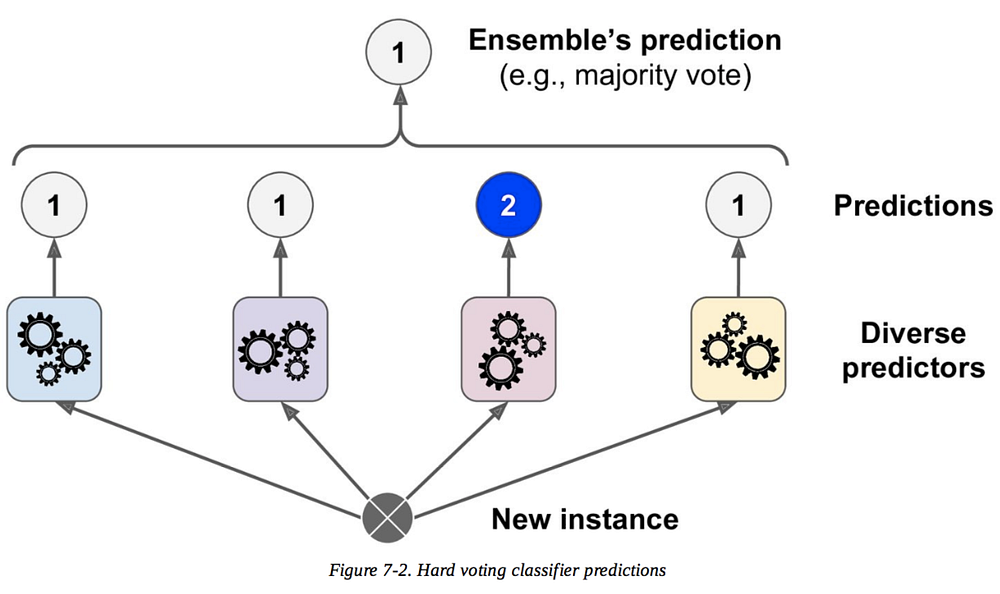“Hands-on Machine Learning with Scikit-Learn & TensorFlow”, Chapter 7

• Averaging

The first example we’ve seen is mostly used for classification problems. Now let’s take a look at a technique used for Regression problems known as Averaging. Similar to Hard Voting, we take multiple predictions made by different algorithms and take its average to make the final prediction.

• Weighted Averaging

This technique is equal to Averaging with the twist that all models are assigned different weights according to its importance to the final prediction.

• Stacking

Also known as Stacked Generalisation, this technique is based on the idea of training a model that would perform the usual aggregation we saw previously.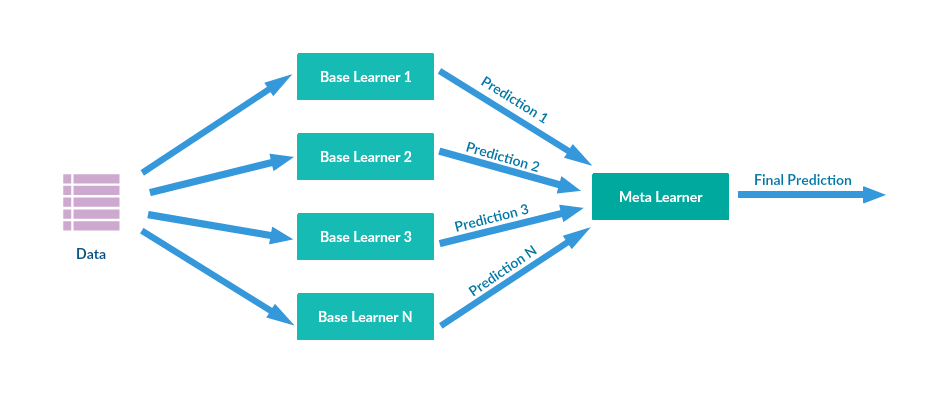We have N predictors, each making its predictions and returning a final value. Later, the Meta Learner or Blender will take these predictions as input and make the final prediction.

Let’s see how it works…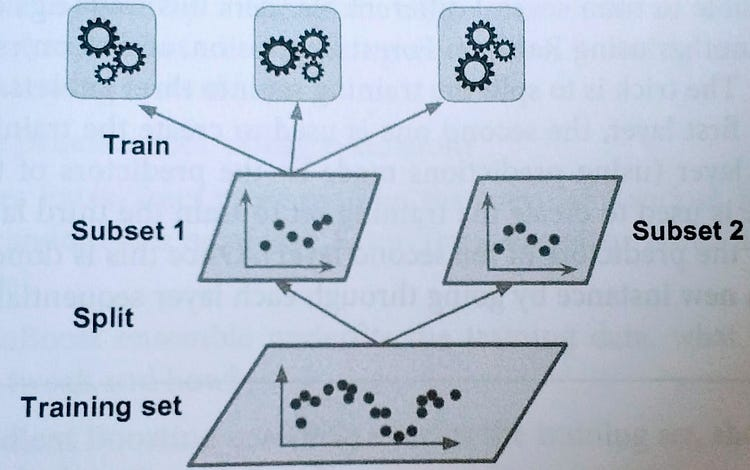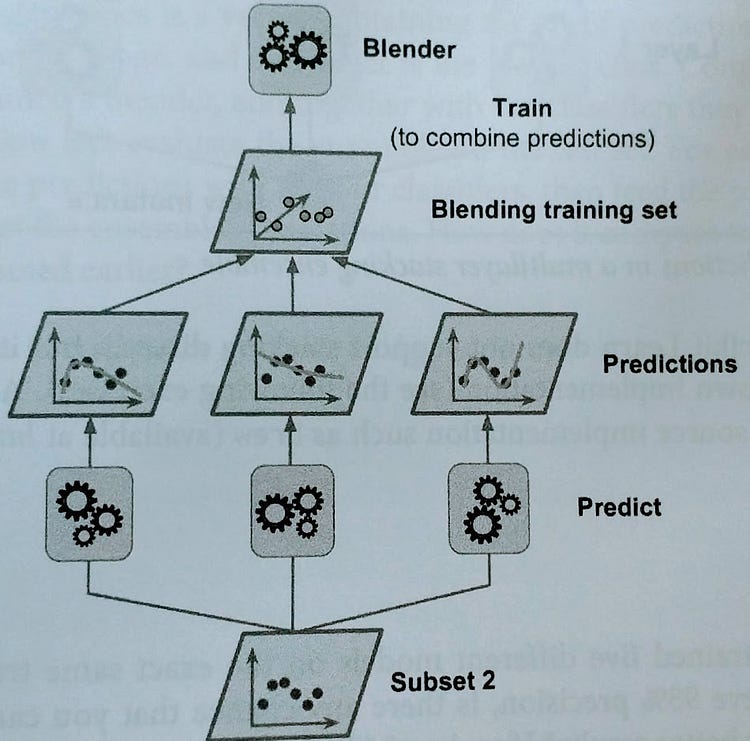Article “Spatial downscaling of precipitation using adaptable random forests”

Regarding the creation of the subsets, you can either proceed with replacement or without. If we assume there is replacement, some observations maybe are present in more than one subset, the reason why we call this method bagging (short for bootstrap aggregating). When the sampling is performed without replacement we ensure that all the observations, in each subset, are unique thus guaranteeing there are no repeated observations in every subset.

Once all the predictors are trained, the ensemble can make a prediction for a new instance by aggregating the predicted values of all trained predictors, just like we saw in the hard voting classifier. Although each individual predictor has a higher bias than if it were trained on the original dataset, the aggregation allows the reduction of both bias and variance (check my post on the bias-variance trade-off)

With pasting, there is a high chance that these models will give the same result since they are getting the same input. Bootstraping introduces more diversity in each subset than pasting, therefore you can expect a higher bias from bagging which means the predictors are less correlated so the ensemble’s variance is reduced. Overall, the bagging model usually offers better results which explains why generally you hear more about bagging than pasting.

• Boosting

If a data point is incorrectly predicted by the first model as well as the next (probably all models), will the combination of their results provide a better prediction? This is where Boosting comes into action.

Also known as Hypothesis Boosting, it refers to any Ensemble method that can combine weak learners into a stronger one. It’s a sequential process where each subsequent model attempts to fix the errors of its predecessor.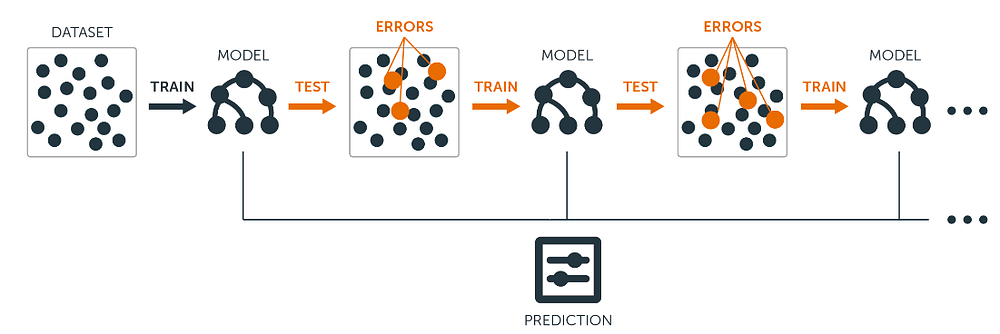Source

Each model would not perform well on the entire dataset. However, they do have great performances in parts of the dataset. Hence, from Boosting we can expect that each model will actually contribute to boosting the performance of the overall ensemble.

Algorithms based on Bagging and Boosting

The most commonly used Ensemble Learning techniques are Bagging and Boosting. Here are some of the most common algorithms for each of these techniques. I’ll write single posts presenting each one of these algorithms in the near future.

Bagging algorithms:

• Random Forest

Boosting algorithms:

• XGBoost
• Light GBM

Bio: Diogo Menezes Borges is a data Scientist with a background in engineering and 2 years of experience using predictive modeling, data processing, and data mining algorithms to solve challenging business problems.

Original. Reposted with permission.

Resources:

• On-line and web-based: Analytics, data Mining, data Science, Machine Learning education
• Software for Analytics, data Science, data Mining, and Machine Learning

Related:

• Intuitive Ensemble Learning Guide with Gradient Boosting
• Improving the Performance of a Neural Network
• A Tour of The Top 10 Algorithms for Machine Learning Newbies